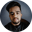Related Tags

solidity
communitycreator

# What is a view function in Solidity?Shubham Singh Kshatriya

In Solidity, a function that only reads but doesn’t alter the state variables defined in the contract is called a View Function.

If the statements below are found in a view function, the compiler will consider them as altering state variables and return a warning.

1. Modify/over-write state variables.
2. Create new contracts.
3. Invoke a function that is not pure or view.
4. Emit event.
5. Using certain opcodes in inline assembly.
6. Use selfdestruct.
7. Use low-level function calls.
8. Send Ether along with function calls.

Note: All the getter functions are view functions by default.

### Syntax

function <function-name>() <access-modifier> view returns() {
// function body
}


### Code

In the code snippet below, we will see how to create a view function in Solidity.

pragma solidity ^0.5.0;

contract Example {
// declare a state variable
uint number1 = 10;

// creating a view function
// it returns sum of two numbers that are passed as parameter
// and the state variable number1
function getSum(uint number2, uint number3) public view returns(uint) {
uint sum = number1 + number2 + number3;
return sum;
}
}

### Explanation

• Line 3: In the above code, we create a contract named Example.

• Line 5: We declare a static variable number1.

• Line 10: We define a view function named getSum().

• Line 11-12: This function accepts two parameters number2 and number3, calculates the sum of number1, number2, and number3, and returns the sum.

Since getSum() is a view function, we can read variable number1 but can’t modify it.

RELATED TAGS

solidity
communitycreator

CONTRIBUTORShubham Singh Kshatriya
RELATED COURSES

View all Courses

Keep Exploring

Learn in-demand tech skills in half the time